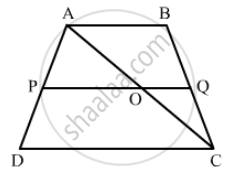Advertisement Remove all ads

# Abcd is a Trapezium in Which Ab || Dc. P and Q Are Points on Sides Ad and Bc Such that Pq || Ab. If Pd = 18, Bq = 35 and Qc = 15, Find Ad. - Mathematics

Sum

ABCD is a trapezium in which AB || DC. P and Q are points on sides AD and BC such that PQ || AB. If PD = 18, BQ = 35 and QC = 15, find AD.

Advertisement Remove all ads

#### Solution

In trapezium ABCD, AB || DC. P and Q are points on sides AD and BC such that PQ || AB.

Join AC. Suppose AC intersects PQ in O.In Δ ACD , OP || CD

∴ (AP)/(PD)=(AO)/(OC).......(1)  (BPT)

In Δ Abc , OQ||AB

∴ (BQ)/(QC)=(AO)/(OC).......(2)  (BPT)

From (1) and (2), we get

(AP)/(DP)=(BQ)/(QC)

(AP)/(18)=(35)/(15)

AP=(35xx18)/(5xx3)

AP=(7xx5xx3xx6)/(5xx3)

AP=(7xxcancel5xxcancel3xx6)/(cancel5xxcancel3)

AP = 42

AD=AP+PD

AD=42+18

AD=60

Hence, the value of AD is  60 .

Concept: Triangles Examples and Solutions
Is there an error in this question or solution?
Advertisement Remove all ads

#### APPEARS IN

RD Sharma Class 10 Maths
Chapter 7 Triangles
Q 14 | Page 126
Advertisement Remove all ads

#### Video TutorialsVIEW ALL 

Advertisement Remove all ads
Share
Notifications

View all notifications

Forgot password?
Course